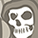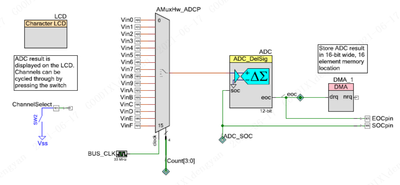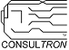PSoC™ 5, 3 & 1Level 1Level 1

# How to resolve the analog multiplexer cross talk for 16 bit ADC ?

Hi

I encountered a problem while using AMUX to switch channel for 16 bit or higher resolution Delsig ADC.

I verify that in CY8CKIT-050 board and run the  example project as below show,  it can works as expected.

but when I change the ADC resolution to 16 bit, and input fixed voltage like half of power, the readout  is  abnormal of which jitter is serious,  I think the Amux cause that, so I increase the delay time before start ADC to keep enough time after change channel, no matter what long I delay, the problem is still exist. I am confused about this problem.

I hope someone can help me solve this problem,  thanks very much!Best Regards,

Ekko

1 SolutionLevel 9Level 9

# Re: How to resolve the analog multiplexer cross talk for 16 bit ADC ?

Ekko,

I've modified your project and simplified it to prove if the the Amux and ADC_DelSig is working at 16bit resolution.

I've attached my modified version of your project.

Here are a list of modifications:

• Disabled all DMA.  The DMA could be a factor in the 'wonky' conversion issues.
• Disabled the DMA_done isr.  This is because I disabled the DMA chain.  I use the ADC_DelSig_IsEndConversion(ADC_DelSig_WAIT_FOR_RESULT).
• I synchronize which channel is being sampled by reading the LUT output through a contiguous port pin assignment.
• Added a UART Terminal debug output for the ADC counts and Converted mV for Vin0, Vin1, and Vin2 inputs. (115.2Kbps 8N1)
• Added a systick ISR to allow for debugging output every 1 second.
• Added 3 VDAC8s to generate static voltages from the CY8CKIT-059 board pins to be routed to the Vin0, Vin1 and Vin2 pins.  This is used as input stimulus without external equipment.
• Changed the VDD values (VDDA, VDDD, VDDIOx etc) in the DWR/System tab to the measured value of 4.8V.  I did this because you chose to use the vref to the ADC_DelSig to be VDDA/4.  Therefore by setting the VDDA value in the DWR/System tab to a more accurate value, allows the ADC_DelSig_CountsTo_mVolts() to better compensate to a more accurate value.   Note:  As /odissey1 pointed out, if you use change the ADC Input range: to "Vssa to 1.25V" and the Reference: Internal 1.024V, this setting is not needed.
• Some pin assignments.  Change if you need.

In the debug output to the terminal, I display Vin0, Vin1 and Vin2 values as ADC counts and converted mV values.

The results are very close to the expected values of Vin0 = 400mV, Vin1 = 100mV and Vin2 = 900mV.

``````Vin0 = 157C cnts   Vin1 = 592 cnts  Vin2 = 3152 cnts    Vin0 = 402mV  Vin1 = 104mV  Vin2 = 924mV
Vin0 = 1579 cnts   Vin1 = 593 cnts  Vin2 = 312F cnts    Vin0 = 402mV  Vin1 = 104mV  Vin2 = 922mV
Vin0 = 157B cnts   Vin1 = 591 cnts  Vin2 = 3108 cnts    Vin0 = 402mV  Vin1 = 104mV  Vin2 = 919mV
Vin0 = 1578 cnts   Vin1 = 592 cnts  Vin2 = 310A cnts    Vin0 = 402mV  Vin1 = 104mV  Vin2 = 919mV
Vin0 = 157A cnts   Vin1 = 596 cnts  Vin2 = 310C cnts    Vin0 = 402mV  Vin1 = 104mV  Vin2 = 919mV
Vin0 = 1571 cnts   Vin1 = 593 cnts  Vin2 = 3111 cnts    Vin0 = 402mV  Vin1 = 104mV  Vin2 = 920mV
Vin0 = 1571 cnts   Vin1 = 593 cnts  Vin2 = 310B cnts    Vin0 = 402mV  Vin1 = 104mV  Vin2 = 919mV
Vin0 = 156D cnts   Vin1 = 593 cnts  Vin2 = 3113 cnts    Vin0 = 401mV  Vin1 = 104mV  Vin2 = 920mV
Vin0 = 1563 cnts   Vin1 = 599 cnts  Vin2 = 3106 cnts    Vin0 = 401mV  Vin1 = 104mV  Vin2 = 919mV``````

Summary:  In this modified project, I can say the ADC_DelSig with front-end AMUX is working.